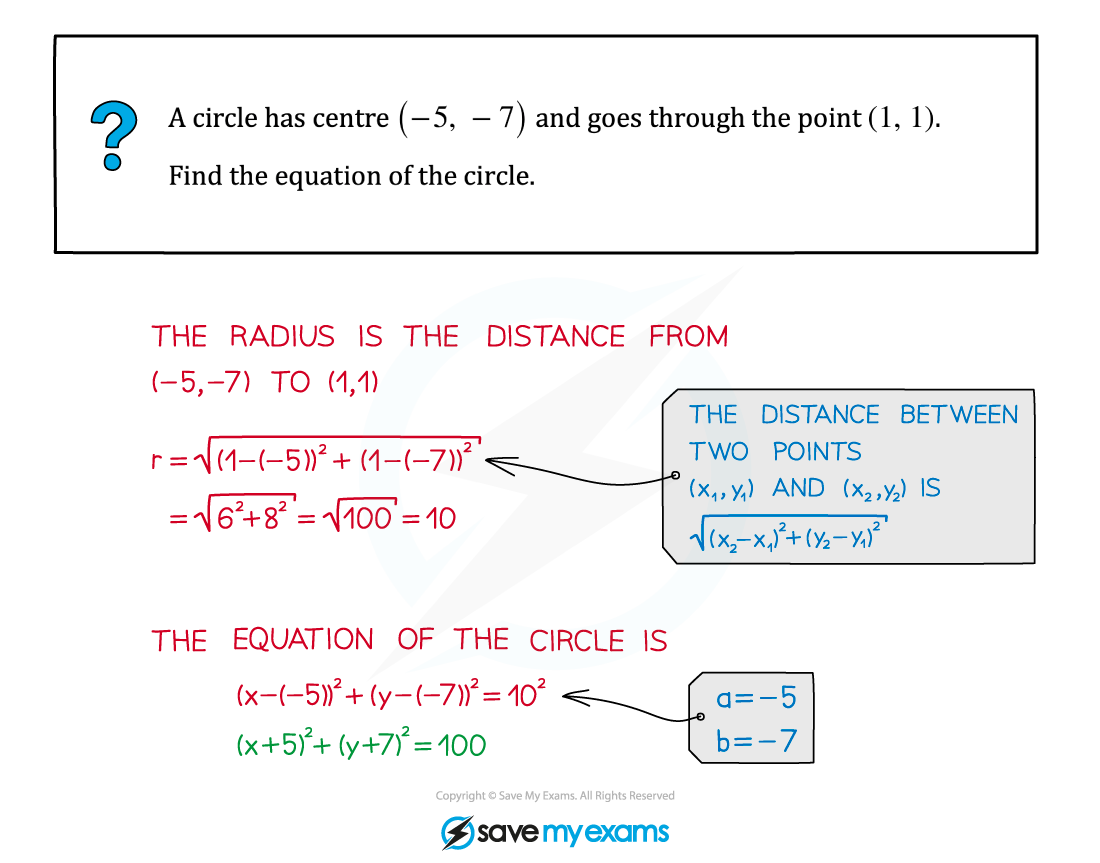# AQA A Level Maths: Pure复习笔记3.2.1 Equation of a Circle

### Equation of a Circle

#### What do I need to know about the equation of a circle?

• A circle with centre (a, b) and radius r has the equation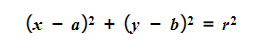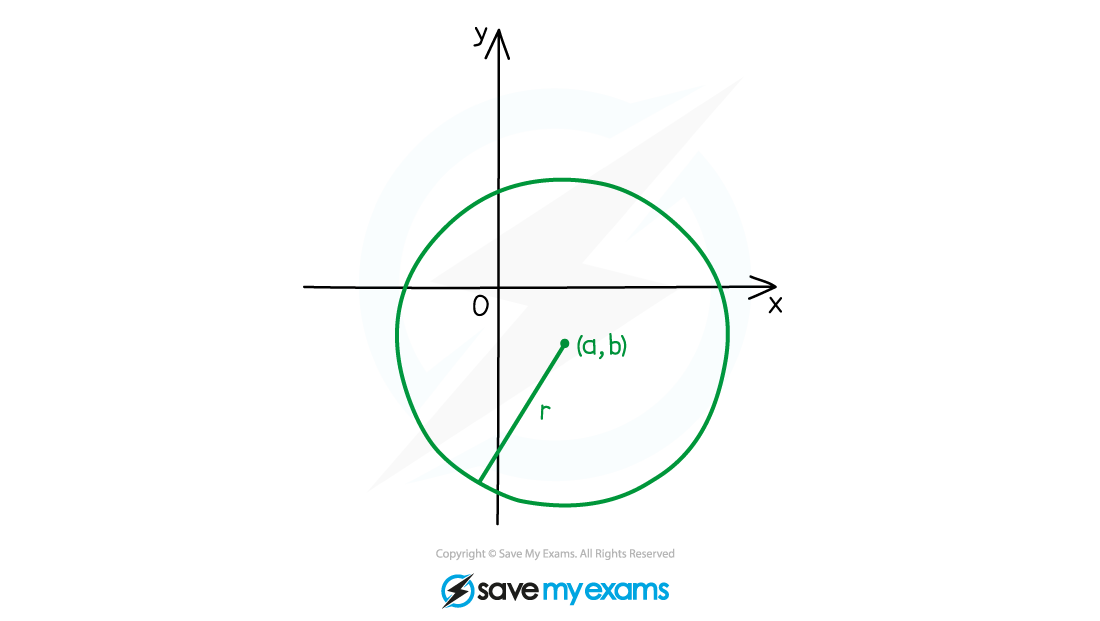•  You need to be able to find the centre and radius of a circle from its equation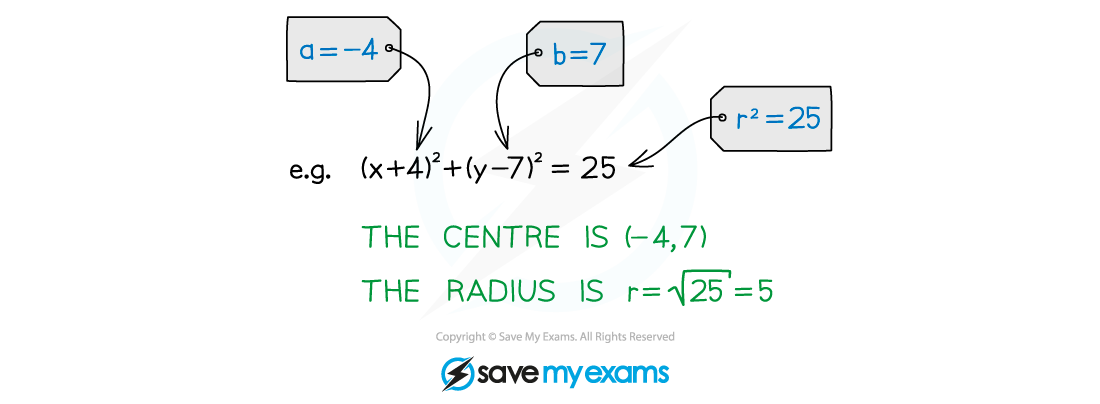•  You need to be able to find the equation of a circle given its centre and radius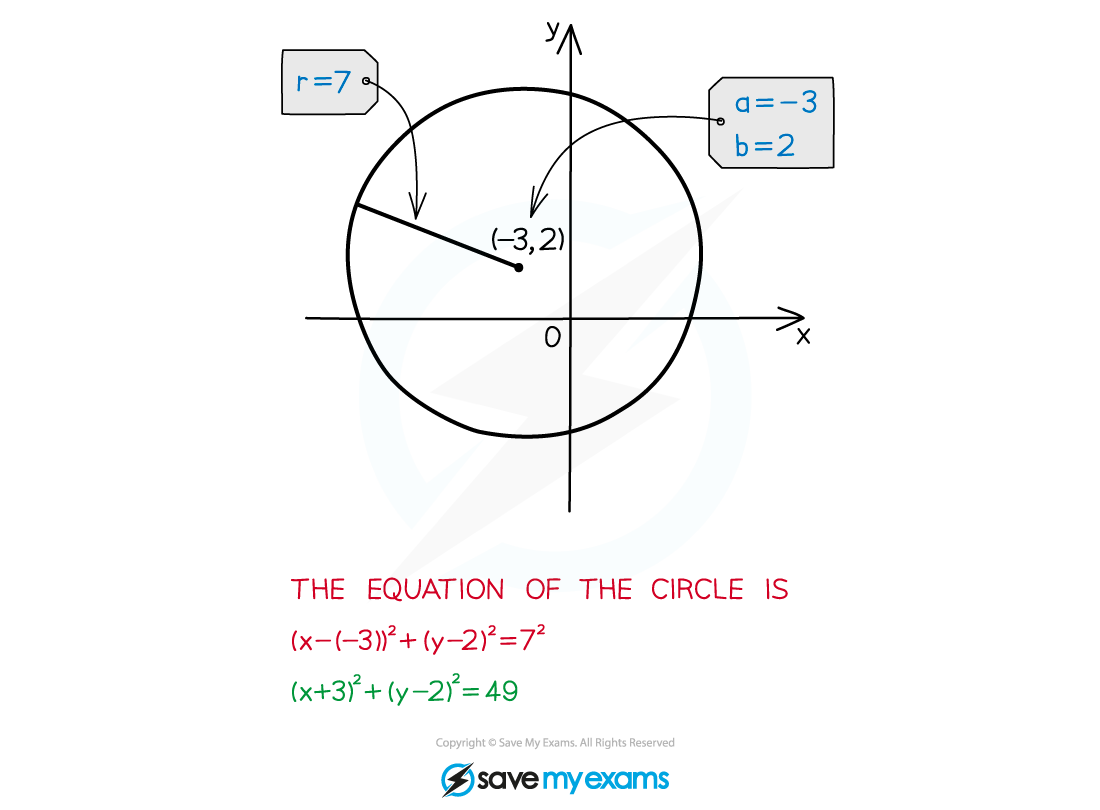#### Exam Tip

• Remember that the numbers in the brackets have the opposite signs to the coordinates of the centre.• Don't forget to take the square root of the right-hand side of the equation when finding the radius.

#### Worked Example